# Insurance Pricing Game : EDA

A brief examination of some metrics that could be considered when modelling.

Hey there! Inspired by this post, here is a notebook with some univariate/bivariate EDA, and a brief examination of some metrics that could be considered when modelling.# Setup the notebook 🛠¶

In [ ]:
!bash <(curl -sL https://gitlab.aicrowd.com/jyotish/pricing-game-notebook-scripts/raw/master/python/setup.sh)
from aicrowd_helpers import *

⚙️ Installing AIcrowd utilities...
Running command git clone -q https://gitlab.aicrowd.com/yoogottamk/aicrowd-cli /tmp/pip-req-build-xns5v9hd
✅ Installed AIcrowd utilities


# Configure static variables 📎¶

In order to submit using this notebook, you must visit this URL https://aicrowd.com/participants/me and copy your API key.

Then you must set the value of AICROWD_API_KEY wuth the value.

In [ ]:
import sklearn

class Config:
TRAINING_DATA_PATH = 'training.csv'
MODEL_OUTPUT_PATH = 'model.pkl'
AICROWD_API_KEY = ''  # You can get the key from https://aicrowd.com/participants/me
'numpy',  # you can define versions as well, numpy==0.19.2
'pandas',
'scikit-learn==' + sklearn.__version__,
]


In [ ]:
# Make sure to offically join the challenge and accept the challenge rules! Otherwise you will not be able to download the data

💾 Downloading dataset...
Verifying API Key...
API Key valid
Saved API Key successfully!


In [ ]:
import numpy as np
import pandas as pd
import matplotlib.pyplot as plt
import seaborn as sns
sns.set()

X_train = df.drop(columns=['claim_amount'])
y_train = df['claim_amount']


# Data Exploration¶

• The dataset provided consists of 228216 - observations corresponding to 57054 unique policies (panel data), with 17 features

• Mixture of numerical and categorical features

• 204924 of these entries are non-claims; the dataset has imbalanced classes

• There is some missing vehicle information: vh_speed, vh_value, vh_weight, vh_age
• drv_age2, drv_age_lic2 depends on having info for second driver
In [ ]:
print(df.shape)
df.count()

(228216, 26)

Out[ ]:
id_policy                 228216
year                      228216
pol_no_claims_discount    228216
pol_coverage              228216
pol_duration              228216
pol_sit_duration          228216
pol_pay_freq              228216
pol_payd                  228216
pol_usage                 228216
drv_sex1                  228216
drv_age1                  228216
drv_age_lic1              228216
drv_drv2                  228216
drv_sex2                  228216
drv_age2                   75320
drv_age_lic2               75320
vh_make_model             228216
vh_age                    228212
vh_fuel                   228216
vh_type                   228216
vh_speed                  225664
vh_value                  225664
vh_weight                 225664
population                228216
town_surface_area         228216
claim_amount              228216
dtype: int64

## Univariate¶

• We can look at the distributions of each feature and the target y, claim_amount
• We can also consider making a new variable claimed=claim_amount > 0, which indicates whether there was a claim
• (We omit vh_make_type as it has over 900 categories, but it may be a useful feature)
In [ ]:
# define categorical and numerical feats
cat_feats = ["pol_coverage","pol_payd","pol_usage","drv_sex1","vh_fuel",
"vh_type","drv_sex2","pol_pay_freq","drv_drv2", "year"] #+ ["vh_make_model"]
num_feats = ["pol_no_claims_discount","pol_duration", "pol_sit_duration","drv_age1",
"drv_age_lic1","drv_age2","drv_age_lic2","vh_age","vh_speed","population",
"town_surface_area","vh_value","vh_weight"]
# partition data
df2 = df.loc[df['claim_amount'] > 0].copy().reset_index(drop=True)
df3 = df.loc[df['claim_amount'] == 0].copy().reset_index(drop=True)

df['claimed'] =df['claim_amount'] > 0

In [ ]:
df[cat_feats].astype(str).describe()

Out[ ]:
pol_coverage pol_payd pol_usage drv_sex1 vh_fuel vh_type drv_sex2 pol_pay_freq vh_make_model drv_drv2 year
count 228216 228216 228216 228216 228216 228216 228216 228216 228216 228216 228216
unique 4 2 4 2 3 2 3 4 975 2 4
top Max No WorkPrivate M Diesel Tourism 0 Yearly rthsjeyjgdlmkygk No 2.0
freq 146516 218696 149976 137868 123940 205496 152896 84850 16724 152896 57054

### Categorical Features¶

Some of the categories have very low counts, so perhaps they could be binned together to reduce the number of categories. The number of observations per year appears to be roughly the same (one thing to do could be to examine whether the distribution of features are approximately constant over the years).

vh_make_type is ommitted as it has over 900 categories

In [ ]:
fig, ax = plt.subplots(ncols=5, nrows=2,figsize=(25, 10))
for i in range(len(cat_feats)):
sns.countplot(x=df[cat_feats[i]], ax =ax[i//5, i % 5])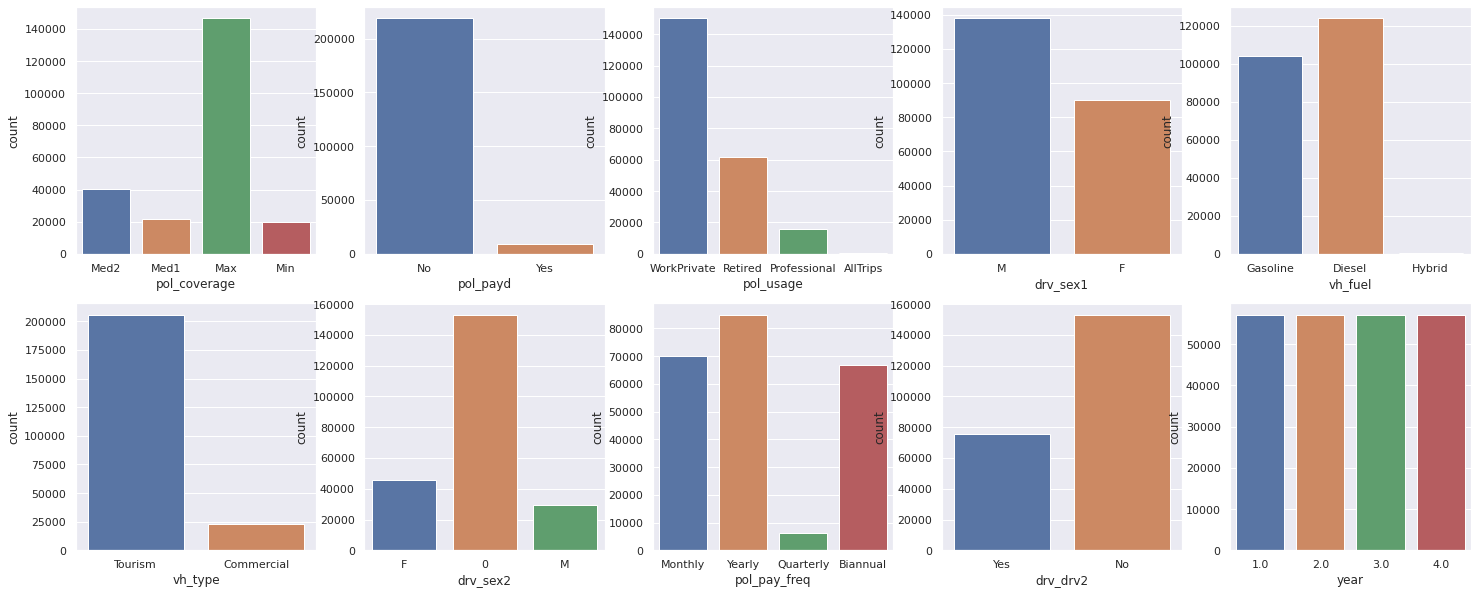### Numerical Features¶

None of the features look normal-like, with the exception of drv_age1, drv_age_lic1, drv_age2, drv_age_lic2 , which suggests a log-transform or power transform, in addition to normalization could be applied

In [ ]:
fig, ax = plt.subplots(ncols=5, nrows=3,figsize=(25, 12.5))
for i in range(len(num_feats)):
sns.histplot(x=df[num_feats[i]], ax =ax[i//5, i % 5])
sns.histplot(df['claim_amount'],ax=ax[2,3])

Out[ ]:
<matplotlib.axes._subplots.AxesSubplot at 0x7f396194ca58>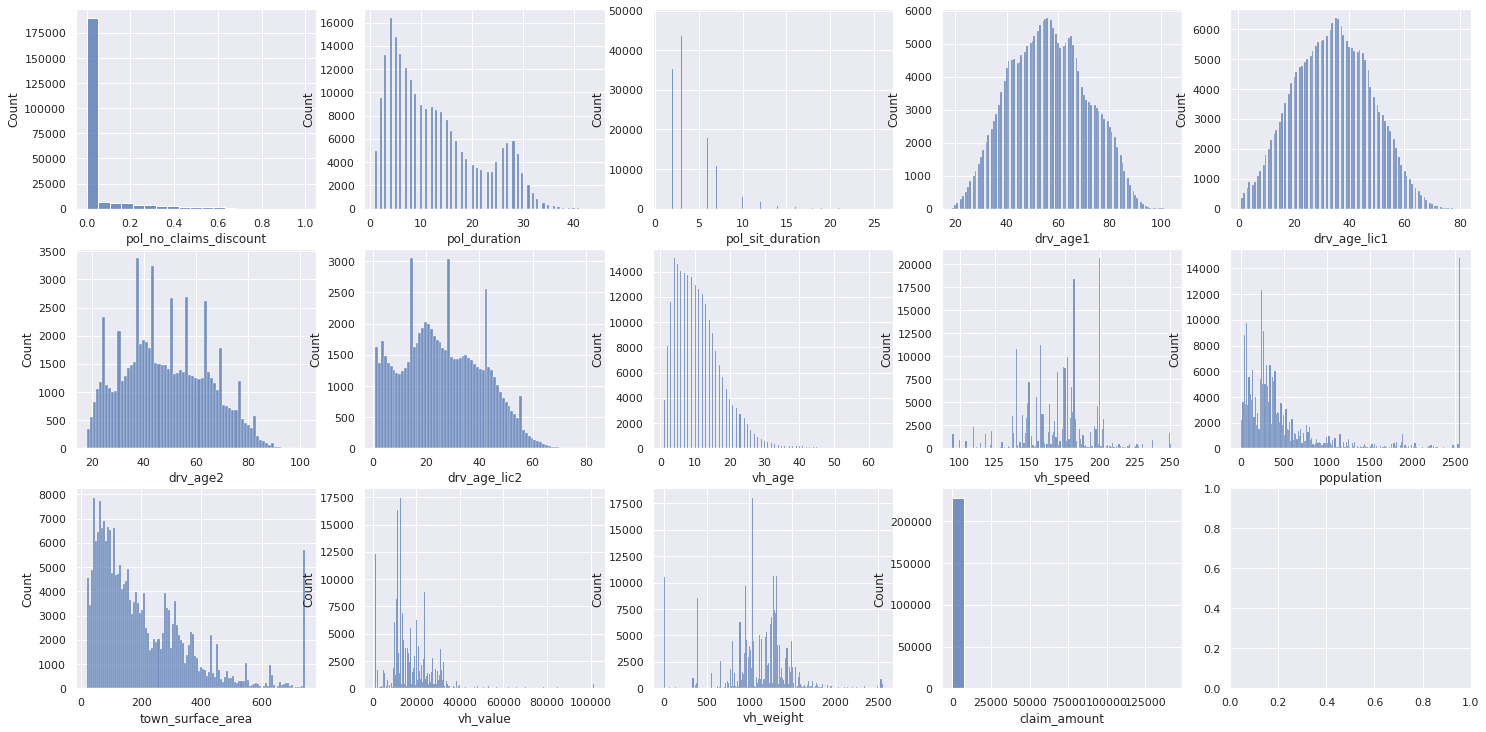the distribution of claim_amount | claim_amount > 0 looks more "normal like" after applying a log transform, suggesting that it may be Gamma-distributed or Log-normally distributed, which could be a consideration for linear models

In [ ]:
sns.histplot(np.log(df2['claim_amount']))

Out[ ]:
<matplotlib.axes._subplots.AxesSubplot at 0x7f395d6b00f0>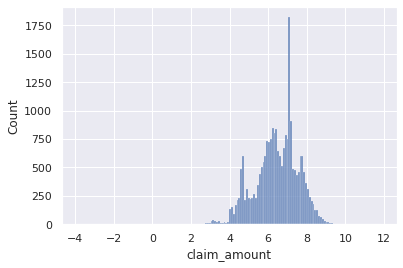## Bivariate¶

### Correlations¶

We can consider the correlations for

• All the features $\mathbf{X}$
• The distribution of features for claim_amount > 0 : $\mathbf{X}|y > 0$

In terms of both Spearman and Pearson correlation, the correlation between the covariates and the targets is low: $|Corr(X_{i}, Y)|< 0.11$.

The Spearman correlation seems higher, which might suggest nonlinear dependence. There are clusters of covariates, which might be a problem for linear models. This may be less of a problem for Tree based models or other nonlinear models, but for tree-based models might suggest the same information (the correlated features) will be used more in the tree construction.

In [ ]:
fig, ax = plt.subplots(figsize=(30,30))
sns.heatmap(df.corr(method='pearson'),annot=True,ax=ax)
ax.set_title("Pearson Correlations",fontsize=30);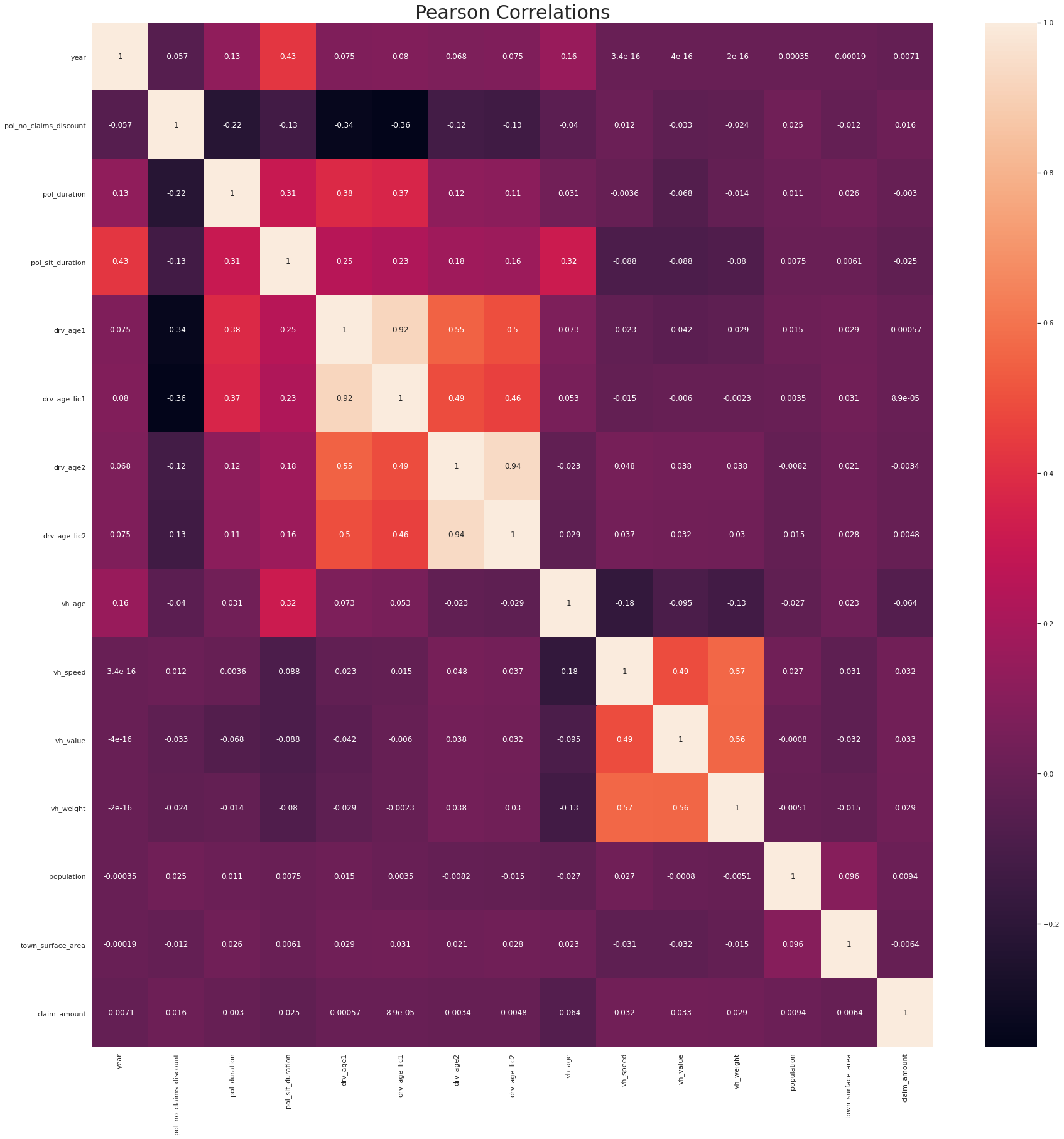In [ ]:
#spearman, all features
fig, ax = plt.subplots(figsize=(30,30))
sns.heatmap(df.corr(method='spearman'),annot=True,ax=ax)
ax.set_title("Spearman Correlations",fontsize=30);

Out[ ]:
<matplotlib.axes._subplots.AxesSubplot at 0x7f396aefcda0>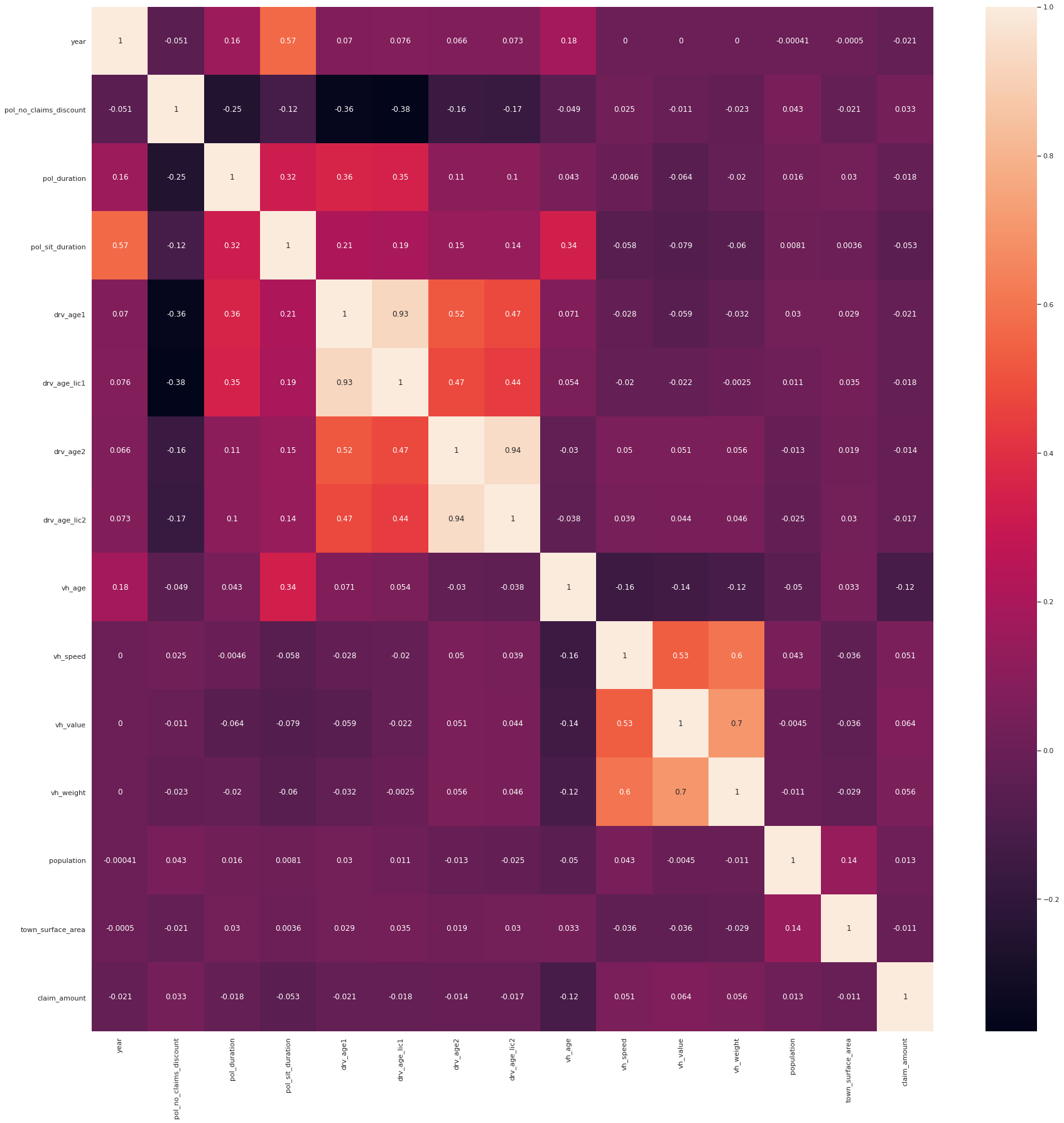In [ ]:
#spearman, all features
fig, ax = plt.subplots(figsize=(30,30))
sns.heatmap(df2.corr(method='pearson'),annot=True,ax=ax)
ax.set_title("Pearson Correlations :Y>0",fontsize=30);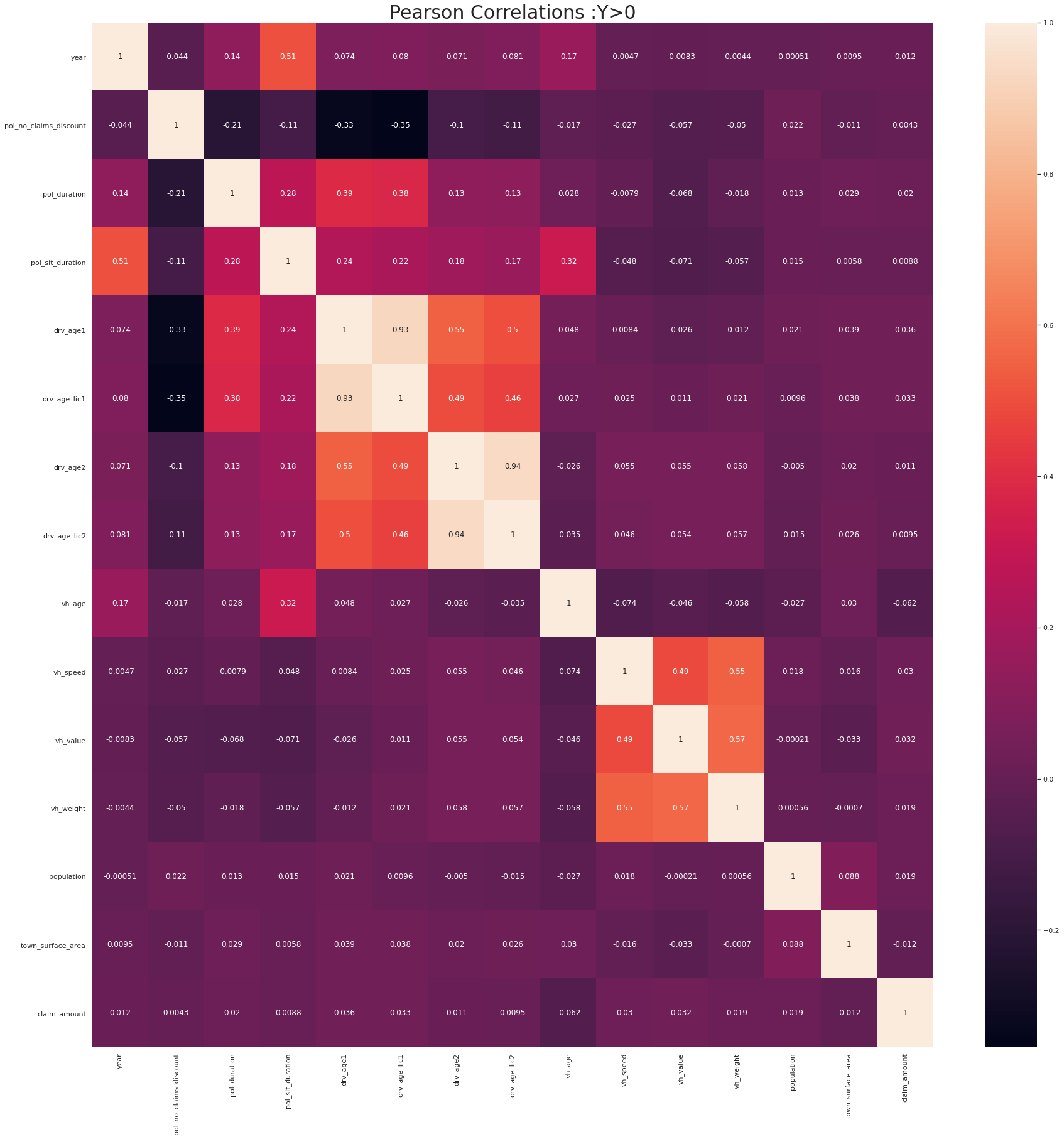In [ ]:
#spearman, all features
fig, ax = plt.subplots(figsize=(30,30))
sns.heatmap(df2.corr(method='spearman'),annot=True,ax=ax)
ax.set_title("Spearman Correlations",fontsize=30);